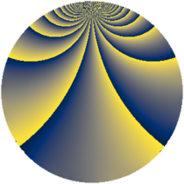# Properties

 Label 3630.2.pLevel $3630$ Weight $2$ Character orbit 3630.p Rep. character $\chi_{3630}(239,\cdot)$ Character field $\Q(\zeta_{10})$ Dimension $864$ Sturm bound $1584$

# Related objects

## Defining parameters

 Level: $$N$$ $$=$$ $$3630 = 2 \cdot 3 \cdot 5 \cdot 11^{2}$$ Weight: $$k$$ $$=$$ $$2$$ Character orbit: $$[\chi]$$ $$=$$ 3630.p (of order $$10$$ and degree $$4$$) Character conductor: $$\operatorname{cond}(\chi)$$ $$=$$ $$165$$ Character field: $$\Q(\zeta_{10})$$ Sturm bound: $$1584$$

## Dimensions

The following table gives the dimensions of various subspaces of $$M_{2}(3630, [\chi])$$.

Total New Old
Modular forms 3360 864 2496
Cusp forms 2976 864 2112
Eisenstein series 384 0 384

## Trace form

 $$864q + 216q^{4} - 4q^{9} + O(q^{10})$$ $$864q + 216q^{4} - 4q^{9} - 8q^{15} - 216q^{16} + 44q^{25} + 30q^{30} + 28q^{31} - 8q^{34} - 16q^{36} - 20q^{39} + 10q^{40} - 48q^{45} + 20q^{46} - 96q^{49} + 20q^{51} + 8q^{60} + 40q^{61} + 216q^{64} + 72q^{69} - 14q^{70} + 48q^{75} + 20q^{79} - 84q^{81} + 20q^{84} + 60q^{85} + 90q^{90} - 24q^{91} + 80q^{94} + O(q^{100})$$

## Decomposition of $$S_{2}^{\mathrm{new}}(3630, [\chi])$$ into newform subspaces

The newforms in this space have not yet been added to the LMFDB.

## Decomposition of $$S_{2}^{\mathrm{old}}(3630, [\chi])$$ into lower level spaces

$$S_{2}^{\mathrm{old}}(3630, [\chi]) \cong$$ $$S_{2}^{\mathrm{new}}(165, [\chi])$$$$^{\oplus 4}$$$$\oplus$$$$S_{2}^{\mathrm{new}}(330, [\chi])$$$$^{\oplus 2}$$$$\oplus$$$$S_{2}^{\mathrm{new}}(1815, [\chi])$$$$^{\oplus 2}$$